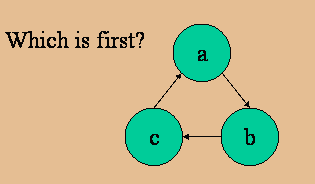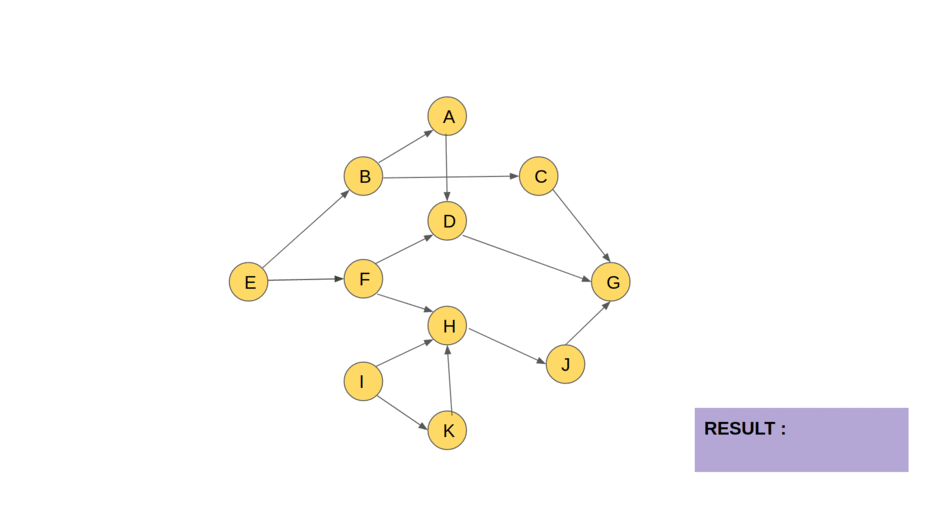# Course Schedule

## Introduction

Task scheduling problems are pretty prominent in the field of programming. In this article, we’ll learn how to solve one such problem named Course Schedule

These problems are frequently asked in Amazon, Microsoft and other product based companies.

Let’s understand the problem statement more precisely in the following section.

## Problem Statement

You have been given ‘N’ courses and some courses may have prerequisites. Now consider a matrix ‘PREREQUISITES’ of size 'M' x 2 which means that you must complete course 'PREREQUISITES[i]' before the course 'PREREQUISITES[i]'.

Your task is to return the ordering of courses you should take to finish all courses.

Note: If there are multiple correct orders, print any one of them. If it is impossible to finish all the tasks, return an empty array.

For example:

## Solution Approach

The base of the solution is to think of this problem as a graph problem where the tasks are the vertices, and the prerequisites are the edges. If task u is a prerequisite of task v; we’ll add a directed edge from u to v.

Now, you must have noticed that this problem reduces to just finding the correct topological ordering of the vertices of the above-described graph. For finding the topological order, we’ll use Kahn’s algorithm. You can learn more about this algorithm here.

Okk before we proceed, what do you think is it possible to find topological ordering in cyclic graphs?Source: giphy

Consider the below cyclic graph and try to find the correct topological ordering.Source: FSU computer science

Yes, you guessed it right. In cyclic graphs you can't decide which one to choose first.

Thus cyclic graphs never have any topological ordering, so if the graph contains a cycle, we’ll return an empty array in that case.

### Stepwise Explanation

• Input the number of tasks (vertices) and edges.
• Store the edges in the adjacency vector and also store the indegree of all the tasks.
• Whenever there is any pre-requisite of task u, increment its indegree by one because there will be an edge incoming towards it due to that prerequisite.
• Create a queue for storing the vertices which don’t need any pre-requisite at the moment.
• Initially, fill the queue with all the vertices whose in-degree is 0.
• Run a loop from 0 to vertices-1 so that all the vertices are there in the topological ordering. Inside that loop, each time, check if the queue is empty.
• If the queue is empty at any iteration, it means that there is a cycle. So just return an empty array.
• Otherwise, take the topmost element of the queue and complete that task. Since we’re finishing it, we’ll be storing it in our v vector, which keeps the topological ordering.
• Since we’re completing the current task, reduce the indegrees of all the tasks by one whose prerequisite was this current task. If, after doing so, there is any task whose indegree gets equal to 0, push it in the queue.
• After doing total “vertices” iterations, return the vector v as it will contain the ordering.
• Finally, print the ordering.

Consider the following visualisation for a better understanding:Source: opengenus.org

Input

Output

### Complexities

#### Time complexity

O(V+E), where V is the number of vertices and E is the number of edges.

Reason: Because there is a for loop running V times and inside it, there is a loop running for edges, and the total number of edges is E.

#### Space complexity

O(V+E), where V is the number of vertices and E is the number of edges.

Reason: V for storing the vertices in the queue and E for storing the edges in the adjacency vector.

1. What is Kahn’s algorithm?

Answer: It is an algorithm used to find the topological ordering of vertices of a graph.

2. Is topological sorting BFS or DFS?

Answer: Topological sorting can be done using both BFS and DFS. However, Khan’s algorithm uses BFS.

3. What is topological sorting?

Answer: Topological sorting is a linear ordering of vertices of a graph such that for every directed edge from u to v, u comes first in the ordering.

4. What is the time complexity of Kahn’s algorithm?

Answer: The time complexity of Kahn’s algorithm is O(V+E), where V is the number of vertices and E is the number of edges.

5. Where can I submit my “Course schedule” code?

Answer: You can submit your code on CodeStudio here and get it accepted right away.

6. Are there more Data Structures and Algorithms problems in CodeStudio?

Answer: Yes, CodeStudio is a platform that provides both practice coding questions and commonly asked interview questions. The more we’ll practice, the better our chances are of getting into a dream company of ours.

## Key takeaways

In this article, we’ve discussed a problem that required topological ordering to be found out. One of the ways to find out the topological ordering was using Kahn’s algorithm. You can also find it out using DFS. For more details on this, refer to this article here. Also, there are many problems related to topological sorting like :

I would suggest you solve them to gain more confidence on this topic. These questions are asked during various coding contests as well as placements tests.

To practice more such problems, Codestudio is a one-stop destination. This platform will help you acquire effective coding techniques and give you an overview of student interview experience in various product-based companies.

Happy Coding!